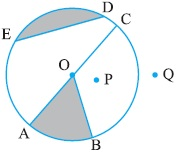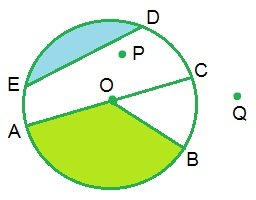Study Materials

# NCERT Solutions for Class 6th Mathematics

Page 6 of 6

## Chapter 4. Basic Geometrical Ideas

### Exercise 4.6

Q1. From the figure, identify :(a) the centre of circle

Solution: The centre of circle is O.

Solution: Three radii are OA, OB and OC.

(c) a diameter

Solution: AC is diameter of circle.

(d) a chord

Solution: ED is a chord.
(e) two points in the interior

Solution: Two points in the interior are P and O.

(f) a point in the exterior

Solution: Q is the point in the exterior of circle.

(g) a sector

Solution: AOB forms sector.

(h) a segment

Solution: ED forms segment.

Q2. (a) Is every diameter of a circle also a chord?
(b) Is every chord of a circle also a diameter?

Solution:

(a) Yes, every diameter of a circle is also a chord.

(b) No, every chord of a circle is not a diameter.

Q3. Draw any circle and mark

(a) its centre

(c) a diameter

(d) a sector

(e) a segment

(f) a point in its interior
(g) a point in its exterior

(h) an arc

Solution:(a) its centre : O

(c) a diameter : AC

(d) a sector : AOB

(e) a segment : ED

(f) a point in its interior : Point P
(g) a point in its exterior : Point Q

(h) an arc : arch AB

Q4. Say true or false :
(a) Two diameters of a circle will necessarily intersect.
(b) The centre of a circle is always in its interior.

Solution:

(a) True

(b) True

Page 6 of 6

Chapter Contents: